# What is the relationship between velocity, frequency and wavelength?

1. What is the relationship between velocity, frequency and wavelength?
• Calculate the wavelength of sound for frequencies upto 130 kHz at which a bat can hear it. [Take, speed of sound = 344 ms–1]
• Express the audible range of frequencies in terms of time-period, if the velocity of sound in air is 330 ms–1.
1. Relationship between velocity, frequency and wavelength are as follows: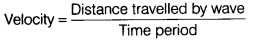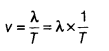…(1)
and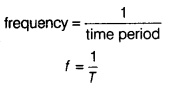⇒ Put the value of f in Eq. (1), we get
Then, ν = λf
velocity = Frequency x Wavelenght
• We know that,
∵ Frequency, f =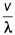Given, velocity of sound in air, v = 344ms–1
Frequency, f = 130 kHz = 130000 Hz
Wavelength, λ =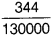[from Eq.(2)]• Audible range of frequencies are, v1 = 20 Hz, v2 = 20000 Hz and
velocity of sound in air, v = 330 ms–1
Time period, (T1) =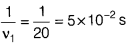T2 =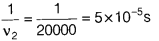Thus, audible range lies between 5 x 10–2s to 5 x 10–5sin terms of time-period.